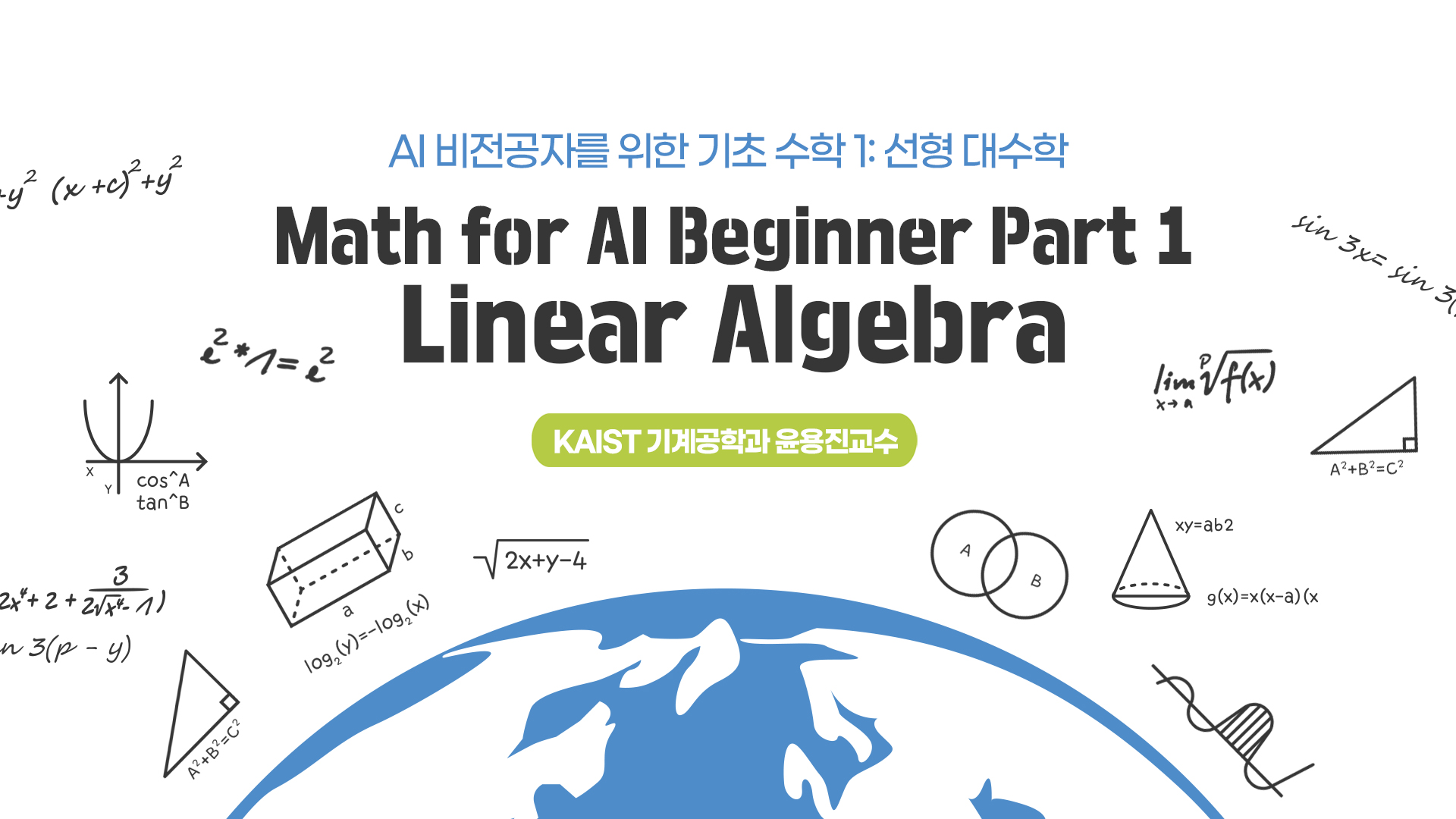## Course summary

• Type MOOC course
• Period Always open
• Learning Time Study freely
• Course approval method Automatic approval
• Certificate Issue Online
http://kooc.kaist.ac.kr/mathforai
Thumb up 780 Learner 1904### Instructor Introduction

•KAIST 기계공학과 윤용진 교수님

KAIST 기계공학과 윤용진 교수님

### Lecture plan

강의
1. Week 1 : Introduction of AI
1. Introduction of AI - 1
1. Introduction of AI - 2
1. Introduction of AI - 3
1. Introduction of AI - 4
1. Introduction of AI - 5
1. Week 1 강의 교안
2. Week 2 : Introduction of Linear Algebra
1. Introduction of Linear Algebra - 1
1. Introduction of Linear Algebra - 2
1. Introduction of Linear Algebra - 3
1. Introduction of Linear Algebra - 4
1. week2-Quiz
1. Week 2 강의 교안
3. Week 3 : Low operation and Linear Combination
1. Row operation and Linear Combination - 1
1. Row operation and Linear Combination - 2
1. Row operation and Linear Combination - 3
1. Row operation and Linear Combination - 4
1. week3-Quiz
1. Week 3 강의 교안
4. CHAPTER 4
1. Linearly independent & inverse of a square matrix-1
1. Linearly independent & inverse of a square matrix-2
1. Linearly independent & inverse of a square matrix-3
1. Linearly independent & inverse of a square matrix-4
1. week4-Quiz
1. Week 4 강의 교안
5. CHAPTER 5
1. Determinant of Square Matrix and Eigenvalue Problem-1
1. Determinant of Square Matrix and Eigenvalue Problem-2
1. Determinant of Square Matrix and Eigenvalue Problem-3
1. Determinant of Square Matrix and Eigenvalue Problem-4
1. week5-Quiz
1. Week 5 강의 교안
6. CHAPTER 6
1. Diagonalisation proberm & 응용분야-1
1. Diagonalisation proberm & 응용분야-2
1. Diagonalisation proberm & 응용분야-3
1. Diagonalisation proberm & 응용분야-4
1. week6-Quiz
1. Week 6 강의 교안
7. ★강의 수강 후 의견을 부탁드리겠습니다.★
1. 교수님 강의에 대한 별점을 매겨주세요. 여러분의 의견이 많은 도움이 됩니다:D